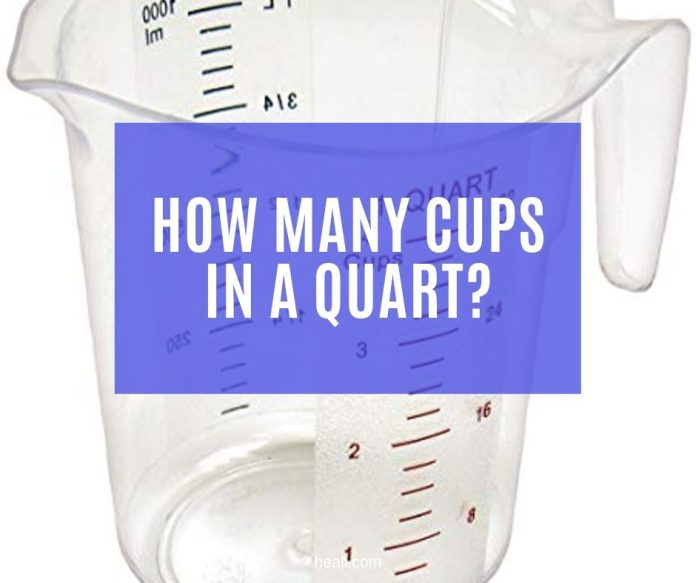# How Many Cups in a Quart?

0
4753Cups and quarts are two of the most basic units of measurement for cooking. Cups are usually used for dry ingredients while the quart is used mainly for liquid ingredients.

Cups are generally used in measuring flour for baking and quart is often used for beer and milk. But how many cups are we referring to when we are talking of a quart? And why is it important for us to know how many cups in a quart?

[CP_CALCULATED_FIELDS id=”33″]

## What is a Cup?

The cup is a unit of measurement for volume capacity. It is expressed using the abbreviation c. For us to better contextualize how one cup looks like, we could equate it to half a pint, 250 mL or ¼ of a litre.

Drinking cups are not good measures of a cup because they vary in size. What we are referring to would be the standard measuring cup used in baking or cooking.

In the US, the standard cup is equivalent to 8 fluid ounces or 16 tablespoons. For the imperial/UK and other commonwealth countries, one cup is equal to 8.80 fluid ounces or 16 and 2/3 tablespoons. In Canada, one cup is equivalent to 8 UK/imperial fluid ounces.

1 cup (US) = 8 fluid ounces (US) = 16 tablespoons (see how many tablespoons in a cup)

1 cup (imperial/UK) = 8.80 fluid ounces = 16 2/3 tablespoons

1 cup (Canada) = 8 imperial fluid ounces

Also read: How many ounces in a cup?

## What is a Quart?

The quart, standardly expressed in the abbreviation qt. is used as a unit of measurement for capacity. Internationally, there are three measurements for quartz depending on what is being measured: the dry quartz which is used for dry ingredients in cooking such as flour and baking soda, liquid quartz used in liquid ingredients such as milk, cooking oil and others and the imperial quartz used for both dry and liquid ingredients in the United Kingdom.

1 quart = 2 pints

1 pint = 2 cups (See how many cups in a pint)

## Quarts To Cups Converter

[CP_CALCULATED_FIELDS id=”33″]

## How many cups in a quart?

If we use the standard conversion value which is aligned with the US metric system, one quart would be composed of 4 cups. But if we use the UK/imperial conversion value, one quart would be equal to 3.33 cups. For us to further convert, all we have to do is to multiply the value of quart to its conversion value to cups. For visuals, you may refer to the table below:

1 quart (US, fluid) = 4 cups (US)

1 quart (US, fluid) = 3.33 cups (UK/imperial)

1 quart (US, dry) = 4.6546 cups (US)

1 quart (UK, fluid) = 4 cups (UK/imperial)

1 quart (US, fluid) = 3.785 cups (metric)

1 quart (UK) = 4.546 cups (metric)

 US quart US cup US quart UK cup 1 4 1 3.33 2 8 2 6.66 3 12 3 9.99 4 16 4 13.32 5 20 5 16.65 6 24 6 19.98 7 28 7 23.31 8 32 8 26.64 9 36 9 29.97 10 40 10 33.3

## How many Canadian cups are in a quart?

1 quart = 5 cups (Canada)

## How many cups is 2 quarts of water?

2 quarts of water = 8 cups

## How many cups in a quart of ice cream?

According to this site called I Love Ice Cream, a quart of ice cream is equal to four cups. But of course, this is the ideal conversion.

In the same site, they mentioned that if we follow the strict conversion and we actually measure one quart of ice cream using a scale, it would only amount to 3.6 cups. But that is not a far conversion value right? So it seems that indeed, one quart of ice cream would be 4 cups.

1 quart of ice cream = 4 cups

## Conclusion

In conclusion, cups to quarts are very important because of two things.

First, cups is versatile and it could be used to both liquid and dry ingredients so when you are using liquid ingredients and you need to express it through cups from quarts, it would come in handy.

Second, there are different values for cups to quarts depending on where you are in the world. We suggest that you follow what the recipe book tells you so never forget that UK quart would amount to 3.33 cups and the standard conversion value using the US metric system is one quart is to 4 cups.